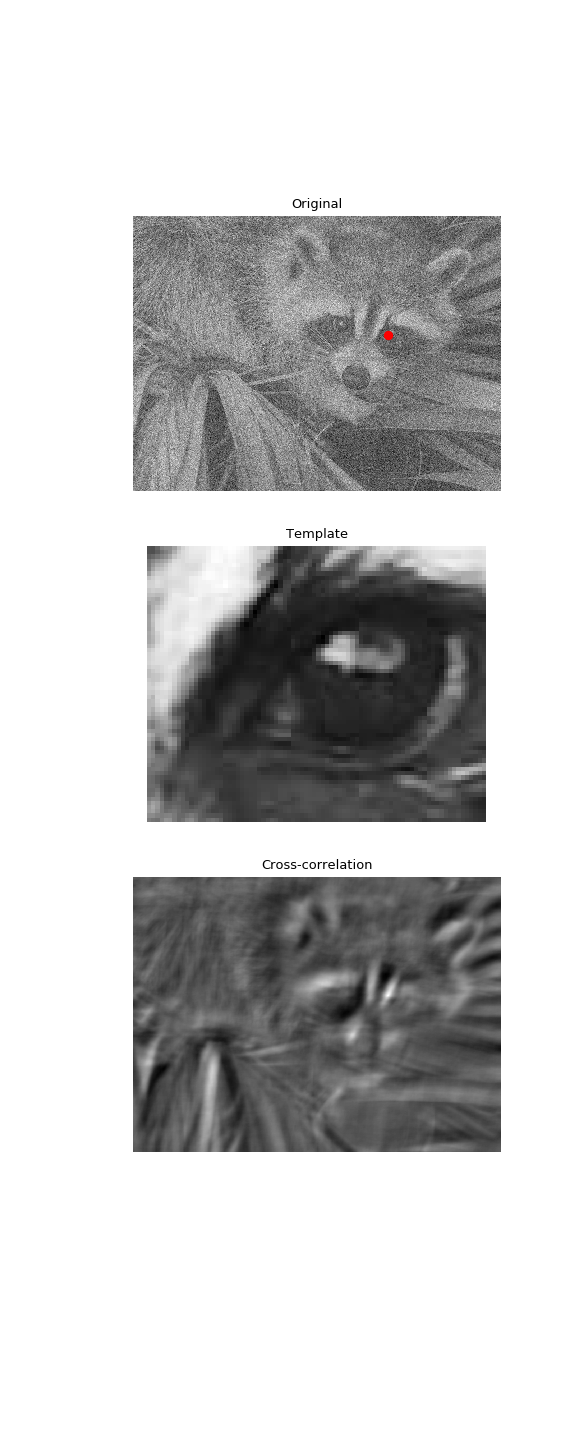# scipy.signal.correlate2d¶

scipy.signal.correlate2d(in1, in2, mode='full', boundary='fill', fillvalue=0)[source]

Cross-correlate two 2-dimensional arrays.

Cross correlate in1 and in2 with output size determined by mode, and boundary conditions determined by boundary and fillvalue.

Parameters: in1 : array_like First input. in2 : array_like Second input. Should have the same number of dimensions as in1. If operating in ‘valid’ mode, either in1 or in2 must be at least as large as the other in every dimension. mode : str {‘full’, ‘valid’, ‘same’}, optional A string indicating the size of the output: full The output is the full discrete linear cross-correlation of the inputs. (Default) valid The output consists only of those elements that do not rely on the zero-padding. same The output is the same size as in1, centered with respect to the ‘full’ output. boundary : str {‘fill’, ‘wrap’, ‘symm’}, optional A flag indicating how to handle boundaries: fill pad input arrays with fillvalue. (default) wrap circular boundary conditions. symm symmetrical boundary conditions. fillvalue : scalar, optional Value to fill pad input arrays with. Default is 0. correlate2d : ndarray A 2-dimensional array containing a subset of the discrete linear cross-correlation of in1 with in2.

Examples

Use 2D cross-correlation to find the location of a template in a noisy image:

>>> from scipy import signal
>>> from scipy import misc
>>> face = misc.face(gray=True) - misc.face(gray=True).mean()
>>> template = np.copy(face[300:365, 670:750])  # right eye
>>> template -= template.mean()
>>> face = face + np.random.randn(*face.shape) * 50  # add noise
>>> corr = signal.correlate2d(face, template, boundary='symm', mode='same')
>>> y, x = np.unravel_index(np.argmax(corr), corr.shape)  # find the match

>>> import matplotlib.pyplot as plt
>>> fig, (ax_orig, ax_template, ax_corr) = plt.subplots(3, 1,
...                                                     figsize=(6, 15))
>>> ax_orig.imshow(face, cmap='gray')
>>> ax_orig.set_title('Original')
>>> ax_orig.set_axis_off()
>>> ax_template.imshow(template, cmap='gray')
>>> ax_template.set_title('Template')
>>> ax_template.set_axis_off()
>>> ax_corr.imshow(corr, cmap='gray')
>>> ax_corr.set_title('Cross-correlation')
>>> ax_corr.set_axis_off()
>>> ax_orig.plot(x, y, 'ro')
>>> fig.show()#### Previous topic

scipy.signal.convolve2d

#### Next topic

scipy.signal.sepfir2d